Everyday Math

# Dissection (Step Up Quiz)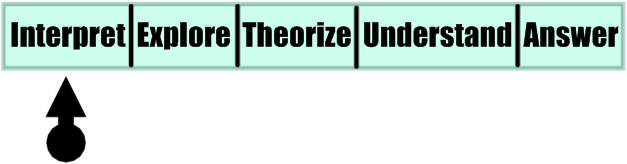This is a series called Step Up which goes through a five-step process of problem solving. Our ultimate goal is to solve the puzzle below.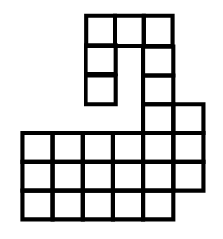Cutting only along the grid lines, divide the figure into two equal parts that are congruent (geometrically the same, rotations and reflections allowed).

Step 1: Analyze the description of the puzzle and understand the conditions.

The figure is composed of 26 squares total. After it is dissected into two parts, how many squares will each individual part have?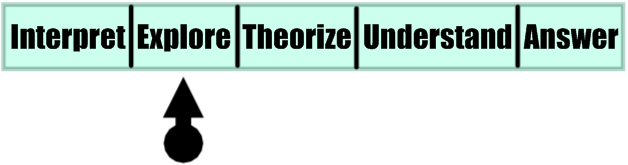This is a series called Step Up which goes through a five-step process of problem solving. Our ultimate goal is to solve the puzzle below.Cutting only along the grid lines, divide the figure into two equal parts that are congruent (geometrically the same, rotations and reflections allowed).

Step 2: Now you should explore and experiment. The "hook" shape looks promising.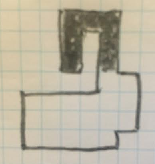The other dissected part must also have the "U" shape. Work out where it should go. Remember rotations and reflections are possible. What must the orientation of the shape be?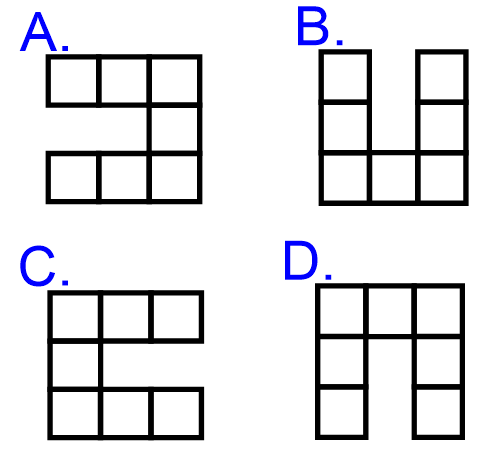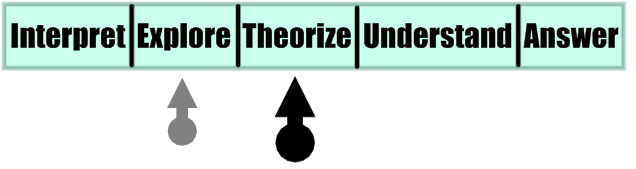Cutting only along the grid lines, divide the figure into two equal parts that are congruent (geometrically the same, rotations and reflections allowed).

Step 3: Using the information from the last step, continue to explore, but also make theories that you can test. With dissection puzzles in particular it's good to pick individual squares and ask which part of the dissection they must go to.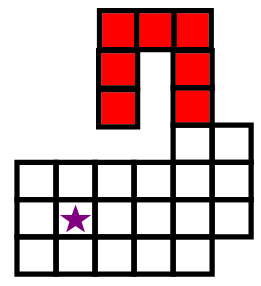Does the red U shape link to the square marked with a star?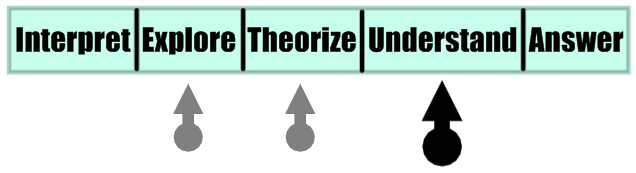Cutting only along the grid lines, divide the figure into two equal parts that are congruent (geometrically the same, rotations and reflections allowed).

Step 4: Keep building theories and you'll soon be close to understanding the problem as a whole.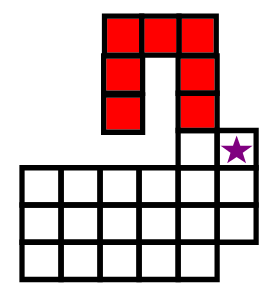Does the red U shape link to the square marked with a star?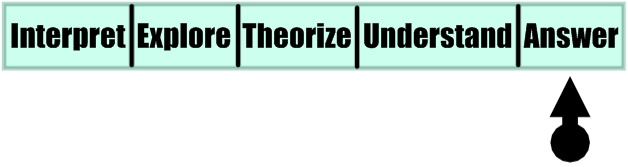Cutting only along the grid lines, divide the figure into two equal parts that are congruent (geometrically the same, rotations and reflections allowed).

Step 5: Time to solve the puzzle!

After you dissect the figure into two equal parts, how long is the boundary between the parts? (Two examples of what this means are below.)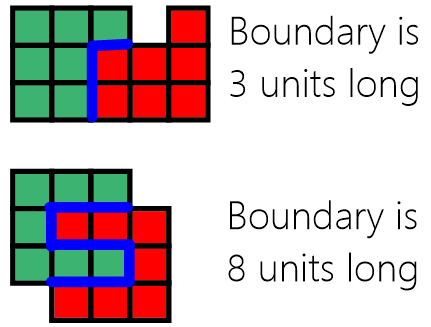×